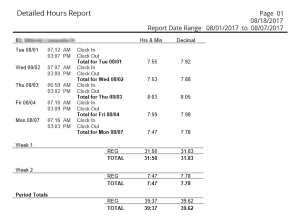# Totaling and Converting Hours and Minutes to Decimal Hours## How to Total & Convert Hours and Minutes to Decimal Hours

Hello again,

We would like to thank Samantha W. at our partner TimeClick® for this guest blog post, and we hope you find this information helpful.

If you are looking for a simpler, faster way to track and calculate employee times, check out TimeClick, an easy, accurate time tracking solution. However, if you don’t have the option to find a new solution yet, the rest of this article will prove useful.

Since time is measured in a base 60 system and money is measured in base 10, you must do a conversion from base 60 to base 10 to find the correct decimal hours for paying your employees.

There are a couple ways to approach the calculation. I will first cover converting an individual day to decimal hours then show totaling all the regular hours on a report at once and converting at the end.

### Getting the Total Regular Hours and Minutes

First, you need to have a total of regular hours and minutes for the day. If an employee clocks in at 7:00 a.m., out at 12:30 p.m. then back in at 1:00 p.m. and out at 3:00 p.m., you’ll need to find the total hours and minutes that they worked.

To calculate the hours for the first time frame we do the following:

1.  Calculate minutes by subtracting the clock in minutes from clock out minutes

:30 – :00 =:30

2.  Calculate the hours by subtracting clock in hour from clock out hour

:12 – 7 = 5

3. Add the two amounts together

:5 +:30 = 5:30

4.  Perform the same calculations for the second clock in and out

:00 – :00 =:00 and 3 – 1 = 2:00

5.  Add the two time frames together

:5:30 + 2:00 = 7:30

We find that this employee worked a total of 7 hours and 30 minutes.

### Converting to Decimal Hours

If you think about it, 7 hours and 30 minutes is not 7.30. 30 minutes is half of an hour (30÷60=.5). If you are adding time cards, you must now convert the regular hours and minutes to decimal hours.

The easiest way to accurately convert regular hours and minutes to decimal hours is to use this formula: Hours + (Minutes ÷ 60).

### What Miscalculations Can Do

Miscalculation can have a large impact in the short and long term if left unchecked. Let’s go through an example together. Let’s say John is paid \$10 per hour and he worked for 5 hours and 00 minutes. That calculation is easy.

\$10 x 5=\$50

But what if John works for 5 hours and 45 minutes? If you do that same calculation replacing the colon in the hours and minutes with a decimal your answer will be

\$10 x 5.45=\$54.50

This would be an incorrect amount of pay for this employee because 45 minutes is not 45 percent of an hour. If you take 45 minutes and divide it by 60 minutes to get the actual percentage, the decimal hour, it would be .75. Plug that into the equation to see the difference.

\$10 x 5.75=\$57.50

The difference between the two calculations is \$3.00. While that seems small now, after 5 days of underpaying the employee by \$3.00 per day, they would be underpaid by \$15. In a year that adds up to \$780 that employee would be underpaid!

### Totaling Time Cards

To approach adding time cards by totaling regular hours and minutes and converting to decimal hours at the end, follow these steps:

Add all the hours together. Add all the minutes. If the total minutes is higher than 60, subtract 60 from the total minutes until the minutes are less than 60. Add the number of subtractions to your hours total. Let’s go through this in more detail to illustrate.

Here is the time sheet the following hours come from. The totals were done automatically by TimeClick.

Click image to view1.       Add the regular hours together:

7 + 7 + 8 + 7 + 7 = 36 hours

55 + 53 + 3 + 59 + 47 = 217 minutes

3.       Subtract 60 from 217 until the total number of minutes is below 60:

217 – 60 = 157 – 60 = 97 – 60 = 37 minutes

4.       Add the number of subtractions to the hours total:

36 Hours + 3 Subtractions of 60 = 39 hours

5.       Convert 39 Hours and 37 Minutes to decimal hours:

39 + (37 ÷ 60) = 39.6166 = 39.62 Hours

The answer for both of these methods, converting one day at a time and adding decimal hours or converting at the end, may be off from the other by .01 or so simply due to rounding but the answers will come out practically the same if done correctly.

If you continue doing these conversions and totals by hand it is highly recommended to have a calculator. The formula is easy. The difficult part is ascertaining there are no errors and taking the time to total and convert all your employee hours by hand.

You may also want to consider using an automated time tracking software to track employee times and total and convert to decimal hours with 100 percent accuracy.

More...

### Partners

Login to our secure portal at the bottom of our Partners' page.

More...